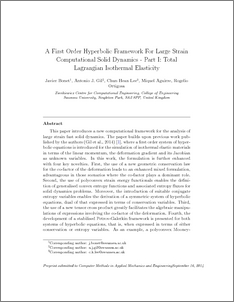# A first order hyperbolic framework for large strain computational solid dynamics. Part I: Total Lagrangian isothermal elasticity

, Gil, A.J.A, Lee, C.H.A, Aguirre, M. A. B. and Ortigosa, R.A. (2015) A first order hyperbolic framework for large strain computational solid dynamics. Part I: Total Lagrangian isothermal elasticity. Computer Methods in Applied Mechanics and Engineering, 283. pp. 689-732. ISSN 0045-7825 (Print), 1879-2138 (Online) (doi:https://doi.org/10.1016/j.cma.2014.09.024)Preview
PDF (Author's Pre-Print Version)
14087_BONET_First_Order_Hyperbolic_2015.pdf - Submitted Version

## Abstract

This paper introduces a new computational framework for the analysis of large strain fast solid dynamics. The paper builds upon previous work published by the authors (Gil etal., 2014) , where a first order system of hyperbolic equations is introduced for the simulation of isothermal elastic materials in terms of the linear momentum, the deformation gradient and its Jacobian as unknown variables. In this work, the formulation is further enhanced with four key novelties. First, the use of a new geometric conservation law for the co-factor of the deformation leads to an enhanced mixed formulation, advantageous in those scenarios where the co-factor plays a dominant role. Second, the use of polyconvex strain energy functionals enables the definition of generalised convex entropy functions and associated entropy fluxes for solid dynamics problems. Moreover, the introduction of suitable conjugate entropy variables enables the derivation of a symmetric system of hyperbolic equations, dual of that expressed in terms of conservation variables. Third, the new use of a tensor cross product  greatly facilitates the algebraic manipulations of expressions involving the co-factor of the deformation. Fourth, the development of a stabilised Petrov-Galerkin framework is presented for both systems of hyperbolic equations, that is, when expressed in terms of either conservation or entropy variables. As an example, a polyconvex Mooney-Rivlin material is used and, for completeness, the eigen-structure of the resulting system of equations is studied to guarantee the existence of real wave speeds. Finally, a series of numerical examples is presented in order to assess the robustness and accuracy of the new mixed methodology, benchmarking it against an ample spectrum of alternative numerical strategies, including implicit multi-field Fraeijs de Veubeke-Hu-Washizu variational type approaches and explicit cell and vertex centred Finite Volume schemes.

Item Type: Article cited By 1 Conservation laws; Entropy variables; Large strain elasticity; Petrov-Galerkin; Polyconvex elasticity; Stabilised finite elements Q Science > QA Mathematics > QA76 Computer softwareT Technology > TA Engineering (General). Civil engineering (General) Vice-Chancellor's Group 20 Apr 2020 16:20 http://gala.gre.ac.uk/id/eprint/14087View Item